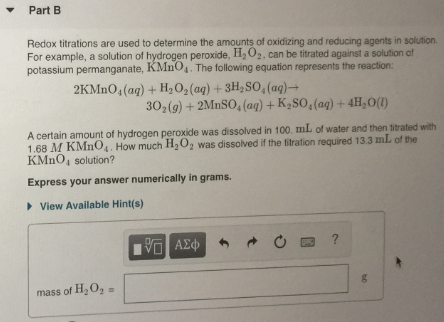# Redox titration are used to determine the amounts of oxidizing and reducing agents in solution. For example, a solution of hydrogen peroxide, H2O2, can be titrated against a solution of potassium permanganate, KMnO4. The following equation represents the reaction: 2KMnO4(aq) + H2O2(aq) + 3H2SO4(aq) → 3O2(g) + 2MnSO4(aq) + K2SO4(aq) + 4H2O(l). A certain amount of hydrogen peroxide was dissolved in 100 mL of water and then titrated with 1.68 M kMnO4. How much H2O2 was dissolved if the titration required 13.3 mL of the KMnO4 solution? Express your answer numerically in grams.